Courses

# Biaxial Bending Civil Engineering (CE) Notes | EduRev

## Civil Engineering (CE) : Biaxial Bending Civil Engineering (CE) Notes | EduRev

The document Biaxial Bending Civil Engineering (CE) Notes | EduRev is a part of Civil Engineering (CE) category.
All you need of Civil Engineering (CE) at this link: Civil Engineering (CE)

Biaxial Bending
» R/C columns under biaxial bending
o The problem of columns under biaxial bending is nonlinear and the number of unknowns is large. For any defined column the problem may be expressed as: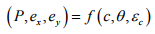where f = a nonlinear function of the variables that can be derived from the equilibrium equations and geometry of any given column section and the stress-strain curves of the materials,
P = axial load, ,
ex, ey = eccentricities measured parallel to x and y axes, respectively,
θ = inclination of neutral axis, c = depth of neutral axis measured from extreme compressive fiber, and
εc = failure strain of concrete in compression.

o A number of approximate methods which are based on simplifying assumptions have been developed. However, for certain situations, the simplifying assumptions may lead to inaccurate results, and the use of presently available design charts is often limited.
o The use of computers to solve this problem with improved accuracy has been based upon iterative analysis of trial sections until a satisfactory result is achieved
o Apart from these considerations, the design process should not be limited to a purely numerical evaluation of the loads, stresses, and strains involved; basic design issues, such as seismic requirements, architectural preferences, availability of material types and sizes, economy, and constructability must be taken into account.

» Basic concept of analysis
o The analysis is based on the strain compatibility and equilibrium equations for the column section.
o For a given neutral axis position, the strains, stresses, and forces in the steel can be found. The resultant force in the concrete depends on the shape of the stress block.
o Consider the following configuration of column section and its strain distribution,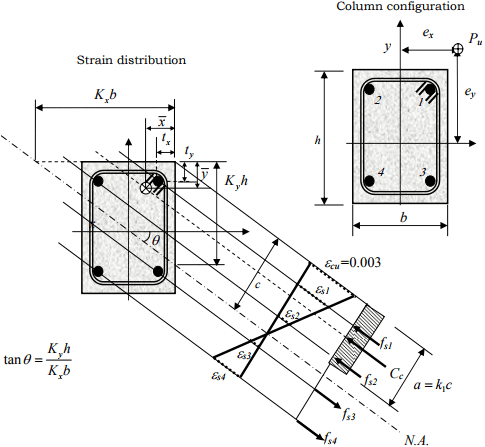Strains in steel bars: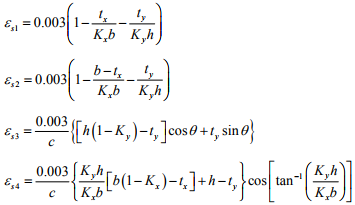o Determination of the stress of steel bars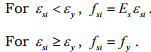o Equilibrium conditions
Forces in steel bars: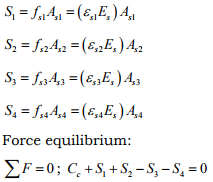Conditions of moment equilibrium are expressed in x and y directions.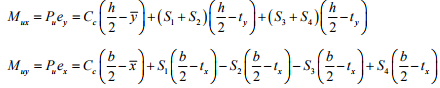Analysis and design requires trials and iterations to find the inclination and depth of the neutral axis satisfying the equilibrium equations.

» Failure interaction curve o The relationship expressed in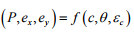describes the three dimensional failure surface (failure interaction curve) if the concrete strain is taken to be εu (usually 0.003). Any combination of neutral axis depth c and inclination θ will give a unique triplet of Pu, eux, and euy corresponding to a point on this failure surface.

o Evaluation of column adequacy using a numerical scheme: In order to declare the adequacy of the column section to resist a given combination of P, ex, and ey only one point on the failure surface need to be computed. Such point satisfies the following condition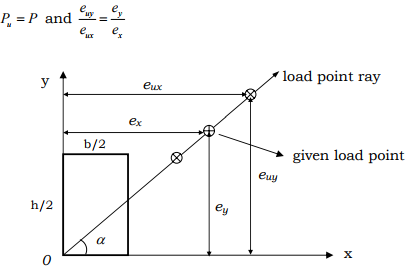→ The procedure is summarized as follows:

(a) Find the neutral axis inclination satisfying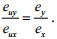(b) Set the neutral axis depth c equal to the neutral axis depth computed from balanced failure condition for the section.
(c) Compute the value of Pu and update c using a modified secant numerical method until Pu =P.
(d) Compute eux and euy and compare with ex and ey to decide whether the section is adequate or not.

o Approximate method for the determination of the neutral axis inclination θ
For rectangular column sections shown: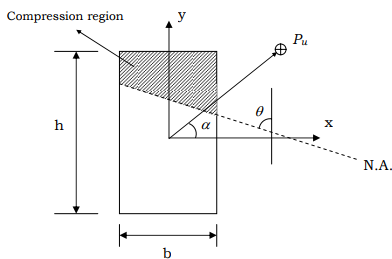Approximately,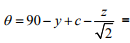inclination of N.A. to y axis

where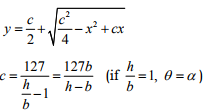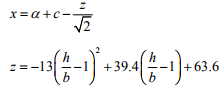» Approximate design methods
o Reciprocal load method: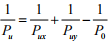where  Pu = ultimate load under biaxial loading,
Pux = ultimate load when only ex is present,
Puy = ultimate load when only ey is present, and
P0 = ultimate load when ex = e= 0 .
o Load contour method: ( Mux − Muy interaction curve in 2D)
For various loads of constant , Pu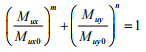where Mux = Puey , Muy = Puex , and Mux0 , Muy0 are uniaxial flexural strengths about x and y axes for the constant load level considered.

→ Experiments suggest that m n = =α depends on column geometry. Typically 1.15 < < α 1.55 for most rectangular columns with uniform reinforcement.
→ There is no single value that can be assigned to the exponent to represent the true shape of the load contour in all cases.

Offer running on EduRev: Apply code STAYHOME200 to get INR 200 off on our premium plan EduRev Infinity!

,

,

,

,

,

,

,

,

,

,

,

,

,

,

,

,

,

,

,

,

,

;